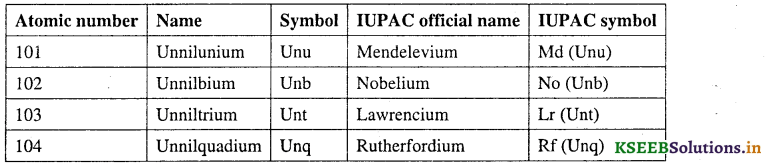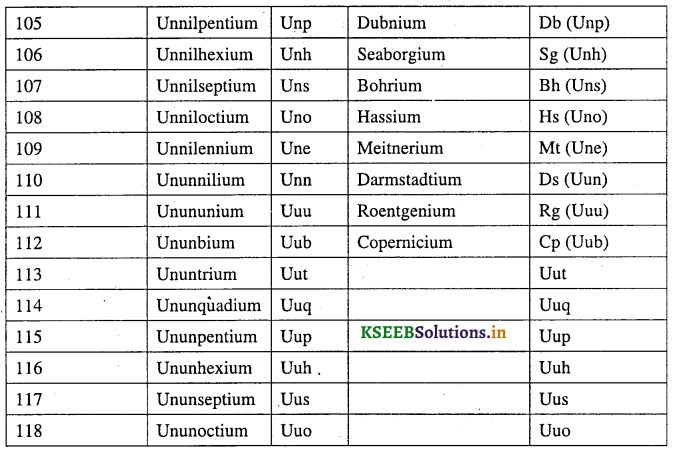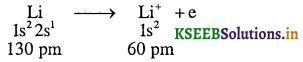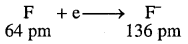# 1st PUC Chemistry Question Bank Chapter 3 Classification of Elements and Periodicity in Properties

Students can Download Chemistry Chapter 3 Classification of Elements and Periodicity in Properties Questions and Answers, Notes Pdf, 1st PUC Chemistry Question Bank with Answers helps you to revise the complete Karnataka State Board Syllabus and to clear all their doubts, score well in final exams

## Karnataka 1st PUC Chemistry Question Bank Chapter 3 Classification of Elements and Periodicity in Properties

Why do We Classify the Elements

Question 1.
Why do we classify the elements systematically?
Systematic classification of elements provides clear idea and information about the properties of elements and makes it easier to predict the properties of elements.

Genesis of Periodic Classification of Elements

Question 1.
Who was the first scientist tried to arrange the elements in the periodic table?
Dobereiner.

Question 2.
Dobereiner proposed the law of triads which states “the atomic mass of the middle element of a triad is nearly equal to the arithmetic mean of atomic masses of the other two elements”.Question 3.
Elements A, B and C constitute a Dobereiner triad. What is the relationship in their atomic mass?Question 4.
For the triad of elements A, B and C, if the atomic weights of A and C are 7 and 39. Predict the atomic weight of B.
Atomic weight of B = $$\frac{7+39}{2}=\frac{46}{2}=23$$

Question 5.
Who stated law of octaves?
Newland.

Question 6.
State law of octaves.
“Every eighth element has property similar to the first element when placed (arranged) in increasing order of their atomic weight.

Question 8.
How many elements were included in the arrangement given by Newland?
8 elements.

Question 7.
State Mendeleev periodic law.
“The properties of the elements are a periodic functions of their atomic weights”.

Question 8.
On what basic did Mendaleev arrange the elements in his period table?
Atomic weight.

Question 9.
What is the predicted name of an element by Mendeleev who left the gap below aluminium?
Eka-aluminium – later it is named as Gallium.

Question 10.
What is the predicted name of an element by Mendeleev who left the gap below silicon?
Eka-silicon – later it is named as Germanium.Modem Periodic Law and the Present form of the Periodic Table

Question 1.
What did Henry Moseley showed by the plot of √v against atomic number of element?
Atomic number of element is the more fundamental property of element than its atomic mass.

Question 2.
State Modern periodic law.
“The physical and chemical properties of the elements are periodic functions of their atomic numbers”.

Question 3.
What are periods in the long form of the periodic table?
The 7 horizontal rows in the periodic table are called periods.

Question14.
What are groups in the periodic table?
The 18 vertical columns of the periodic table are called groups.

Question 5.
How many periods and groups are present in the Iongform of the periodic table?
18 groups and 7 periods.

Question 6.
How many elements are present in each period of the periodic table?
First period – 2 elements.
Second period – 8 elements.
Third period – 8 elements.
Fourth period – 18 elements.
Fifth period – 18 elements.
Sixth period – 32 elements.
Seventh period – 30 elements (incomplete period)

Which quantum number corresponds to the period number in the modern periodic table?
Principal quantum number (n)

Question 7.
On the basis of quantum numbers, how many elements can accommodate in the seventh period (Incompleted period)
32 elements.

Question 8.
How would you justify the presence of 18 elements in the 5th period of the Periodic Table?
When n = 5,l = 0, 1,2, 3. In this period, 5s, 4d and 5p orbitals get filled. The order in which the energy of the available orbitals vary is 5s < 4d < 5p. The total number of orbitals available are 9. The maximum number of electrons that can be accommodated is 18; and therefore 18 elements are there in the 5th period.

What is the basic difference between the Mendeleev’s periodic law and the modern periodic law?
Mendeleev periodic law is based on atomic weight whereas modem periodic law is based on atomic number.Nomenclature of Elements with Atomic Numbers >100

Question 1.
Give the nomenclature of elements with atomic number greater than 100.Question 2.
What would be the IUPAC name and symbol for the element with atomic number 120?
According to IUPAC, the roots for 1, 2 and 0 are un, bi and nil, respectively. Hence, the symbol and the name respectively are Ubn and unbinilium.

Question 3.
The elements Z = 117 and 120 have not yet been discovered. In which family/group would you place these elements and also give the electronic configuration in each case.
The element with atomic number Z = 117 would belong to the halogen family (Group 17) due to the electronic configuration [Rn] 5j146d107s27p5. The element with Z = 120, will be placed in Group 2 (alkaline earth metals), and will have the electronic configuration [Uuo] 8s2.

Electronic Configurations of Elements and the Periodic Table

Question 1.
What is electronic configuration of elements?
The distribution of electrons in various orbitals of an atom is called electronic configuration.

Question 2
Give the classification of elements on the basis of electronic configuration.
On the basis of electronic configuration, elements of the periodic table are classified into four blocks.

1. s-block elements
2. p-block elements
3. d-block elements
4. f-block elementsQuestion 3.
What are s-block elements? Write their valence shell electronic configuration and position in the periodic table.
The elements of group 1 and group 2 of the periodic table are called s-block elements. The general valence shell electronic configuration of s-block elements is ns1-2 (ns1 for group – 1 and ns2 for group – 2). These elements are placed in extreme left of the periodic table.

Question 4.
What are alkali and alkaline earth metals?
The elements of group – 1 (ns1) are called alkali metals and group – 2 (ns2) are called alkaline earth metals.

Question 5.
Explain the position of hydrogen in the periodic table.
Hydrogen has 1s1 electronic configuration. Hence it is placed in group – 1 along with alkali metals. But it can also gain one electron to achieve noble gas configuration and hence it can behave similar to a group – 17 (halogens). Hence hydrogen is placed separately at the top of the periodic table.

Question 6.
Helium should be the s-block element as per the electronic configuration but it is placed in the p-block along with the noble gases. Why.
Because He has completely filled 1s2 configuration and as a result, it exhibits the properties of other noble gases.

Question 7.
Give the general characteristic properties of s-block elements.

1. They are all reactive metals with low ionisation enthalpies.
2. Their outer most electron readily lose to form + 1 ion in alkali metals and + 2 ion in alkaline earth metals.
3. The metallic characters and reactivity increase as we go down the group.
4. They don’t found in nature in the pure state due to high reactivity.
5. The compounds of s-block elements are ionic in nature (except Li and Be compounds).

Question 8.
What are p-block elements? Write their valence shell electronic configuration?
The elements belonging to group 13 to 18 are called p-block elements. The valence shell electronic configuration of p-block elements is ns2np1-6.

Question 9.
What are representative elements?
Both s- and p-block elements are collectively called as representative elements or main group elements.

Question 10.
Group-18 elements are called noble gases. Why?
All group-18 elements have completely filled orbitals and have most stable electronic configuration ns2np6. Therefore, it is difficult to alter this stable arrangement by the addition or removal of electrons. Hence they are called noble gases.

Question 11.
What are halogens?
Group – 17 elements of the periodic table are called halogens, (salt formers)Question 12.
What are chalcogens? (ore formers)
Group – 16 elements of the periodic table are called chalcogens.

Question 13.
What are d-block elements? Write their general valence shell electronic configuration.
The elements from group 3 to 12 at the center of the periodic table are called d-block elements. The valence shell electronic configuration is (n – 1)d1-10 ns1-2.

Question 14.
Give the general characteristic properties of d-block elements.

1. They are all metals.
2. They mostly form coloured ions.
3. They exhibit variable valency (oxidation states).
4. They exhibit paramagnetism.
5. They acts as catalysts.

Question 15.
d-block elements are called transition elements. Why?
The properties of d-block elements are intermediate between s-block and p-block elements. Hence d-block elements are called transition elements.

Question 16.
What are f-block elements? Write their general valence shell electronic configuration.
The two rows of elements at the bottom of the periodic table (Atomic number 58 – 71 and 90 – 103) are called f-block elements.
The general valence shell electronic configuration is (n – 2)f1-14 (n – l)d0-1ns2.

Question 17.
What are lanthanides?
The elements with atomic number 58 – 71 are called lanthanides.

Question 18.
What are actinides?
The elements with atomic number 90 – 103 are called actinides.

Question 19.
f-biock elements are called inner transition elements. Why?
In f-block elements, the last electron added to each element is filled in inner (n – 2) f orbital. Hence they are called inner transition elements.

Question 20.
What are transuranium elements?
The elements after uranium are called transuranium elements.

Question 21.
Explain the classification of elements in the periodic table on their properties.
On the basis of properties of elements, they can be classified into metals, non metals and metalloids.
Metals:

• Metals (more than 78% of all known elements) appear in the left side of the periodic table.
• Metals are usually solids except mercury which is liquid at room temperature.
• Metals usually have high melting points and boiling points (except gallium, mp = 303 K and caesium, mp = 302 K).
• Metals are good conductors of heat and electricity.
• They are malleable (stretched into sheets) and ductile (drawn into wires)

Non metals:

• They are located at the top right hand side of the periodic table.
• Non metals are usually solids or gases at room temperature.
• They have low melting points and boiling points (except boron and carbon)
• They are poor (or bad) conductors of electricity.
• Non metallic solids are brittle and are neither malleable nor ductile.
• Metallic character increases down the group and decreases from left to right in the periodic table.
Metalloids: Some elements like Silicon, Germanium, Arsenic, Antimony and Tellurium shows properties of both metals and non metals are called metalloids or semimetals.

Question 22.
Considering the atomic number and position in the periodic table, arrange the following elements in the increasing order of metallic character: Si, Be, Mg, Na, P.
Metallic character increases down the group and decreases along the period.
Hence increasing metallic character is P < Si < Be < Mg < Na.Periodic Trends in Properties of elements

Question 1.
What is meant by the term periodic trends in properties of elements?
The variation of properties of elements due to their position in the periodic table is called periodic trends.

Question 2.
(a) Atomic radius: The distance from the center of the nucleus to the outermost shell of an atom is called atomic radius.

(b) Covalent radius: It is defined as one-half the distance between the nuclei of two covalently bonded atoms.,

(c) Metallic radius: It is defined as half the inter – nuclear distance separating the metal cores in the metallic crystal.
Note: Practically measurement of atomic radius of isolated atom is very difficult. So, atomic radius refers to both covalent and metallic radius depending on whether the element is a non metal or a metal.

Question 3.
How does atomic radius vary in a period and in a group? How do you explain the variation?
(a) Atomic radius generally decreases across a period.
Explanation: It is because within the period the outer electrons are in the same valence shell. So effective nuclear charge increases as the atomic number increases. Hence atomic size decreases.

(b) Atomic radius increases down the group in the periodic table.
Explanation: This is because within a vertical column of the periodic table, number of shells (principal quantum number) increases. So size of atom increases.

Question 4.
How cations are formed?
Cations are formed by the loss of electrons.
Example: Li → Li+ + e

Question 5.
Size of cation is less than its parent neutral atom. Why?
A cation is formed by the loss of electron. As a result effective nuclear charge increases. So the size decreases.
Example:Question 6.
How anion is formed? Anion size is more than its neutral atom. Why?
Anion is formed when one or more electrons are added to the valence shell of the atom.Thus, anion has more electrons than its parent atom. The added electrons would result in increased repulsion and a decrease in effective nuclear charge. Therefore size of anion is more than its neutral atom.

Question 7.
What are isoelectronic species?
Different species having same number of electrons are called isoelectronic species.
Examples:
O2-, F, Na+ and Mg2+ are isoelectronic species.
O2- = 8e + 2e = 10 electrons
F = 9e + 1e = 10 electrons
Na+ = 11e – 1e = 10 electrons
Mg2+ = 12e – 2e = 10 electrons

Question 8.
Isoelectronic species have different ionic radii. Why?
Because isoelectronic species have different nuclear charges due to the presence of different number of protons (positive charges).

Question 9.
Cation with the greater positive charge will have smaller radius. Why?
Because of the greater attraction of the electrons to the nucleus. Or due to high effective nuclear charge.

Question 10.
Anion with the greater negative charge will have the larger radius. Why?
Because greater repulsion of more number of electrons.Question 11.
Define ionisation enthalpy. How does it vary along the period and down the group in the periodic table?
The amount of energy required to remove an electron from an isolated gaseous atom in the ground state is called ionisation enthalpy.
X(g) → X+(g) + e ΔiH = Q kJ
Ionisation enthalpy increases along the period. This is because atomic size decreases and nuclear force of attraction on outer most electron increases.
Ionisation enthalpy decreases down the group. This is because atomic size increases down the group due to increase number of shells.

Question 12.
Second ionisation enthalpy is greater than first ionisation enthalpy. Explain.
After removing one electron, the effective nuclear charge increases. The remaining electrons are more tightly bounded to the nucleus. So it is very difficult to remove the 2nd electron. Hence 2nd ionisation enthalpy is greater than 1st ionisation enthalpy.

Question 13.
Ionisation enthalpy of alkali and alkaline earth metals is very less. Why?
Due to their large size and high reactivity.

Question 14.
The first ionisation enthalpy of boron is less than beryllium. Give reason.
Electronic configuration of Boron is 1s22s22p1 and Beryllium is 1s22s2. Beryllium has completely filled orbitals. So it is difficult to remove the electron from Beryllium atom.

Question 15.
The first ionisation enthalpy of nitrogen is more than oxygen. Give reasons.
Nitrogen has half filled 2p orbitals (2p1x 2p1y 2p1z). It gives extra stability to the nitrogen atom. But in oxygen atom, two of the four 2p electrons are paired (2p2x 2p1y 2p1z). This results increased electron-electron repulsion. Consequently it is easier to remove the fourth 2p electron in oxygen than nitrogen atom.

Question 16.
Define electron gain enthalpy. How does it vary along the period and down the group of the periodic table?
The amount of energy released during the addition of an electron to a neutral gaseous atom to convert it into a negative ion is called electron gain enthalpy.
X + e → X ΔgH = -QkJ
Electron gain enthalpy may be – ve.
Electron gain enthalpy decreases (more negative) along the period. This is because the effective nuclear charge increases from left to right. Hence it will be easier to add an electron to a smaller atom.

Electron gain enthalpy decreases down the group. This is because size of the atom increases and the added electron would be farther from the nucleus.

Question 17.
The electron gain enthalpy of oxygen or fluorine is less negative than that of the succeeding elements sulphur or chlorine? Explain.
This is because when an electron is added to oxygen or fluorine, the added electron goes to the n = 2 quantum level and suffers significant repulsion from the other electrons present in this level.
But in sulphur or chlorine, the added electron occupies a larger region of space (n = 3 quantum level) and electron-electron repulsion is much less.

Question 18.
Which of the following will have most negative electron gain enthalpy and which the least negative? P, S, Cl, F.
Most negative electron gain enthalpy element is chlorine and less negative electron gain enthalpy element is phosphorus.Question 19.
Halogens (group – 17) have very high negative electron gain enthalpies. Why?
Because halogens can attain stable noble gas electronic configurations by picking up an electron.• Fluorine has less electron gain enthalpy than chlorine.
• Electron gain enthalpy fluctuates in halogens. F < Cl > Br > I.
• Chlorine has highest electron gain enthalpy.

Question 20.
Noble gases have large positive electron gain enthalpies. Why?
Because the electron has to enter the next higher principal quantum level leading to a very unstable electronic configuration.

Question 21.
Define electronegativity.
The ability of an atom in a covalent bond to attract shared pair of electrons towards itself is called electronegativity.
Note:

• Electronegativity is not a measurable quantity. However, a number of numerical scales of electronegativity of elements like Pauling scale, Mulliken – Jaffe scale etc are used.
• Decreasing order of electronegativities is F > O > N.

Question 67.
How does electronegativity varies along the period and down the group? Explain.
In general, electronegativity increases (across a period) and decreases down the group.
This is due to decrease in size along the period and increase in size down the group.

Question 22.
Explain the relation between electronegativity and non metallic character of elements.
Non metallic elements have strong tendency to gain electrons. Hence, electronegativity is directly related to that non metallic properties of elements and inversely related to the metallic properties of elements.

Thus, the increase in electronegativities across a period is accompanied by increase in non-metallic properties (or decrease in metallic properties) of elements. Similarly, the decrease in electro negativity down a group is accompanied by decrease in non-metallic properties (or increase in metallic properties) of elements.

Question 23.
Name the most electronegative element in the periodic table.
Fluorine (F)Question 24.
What are the reasons for the different chemical behaviour (anamolous properties) of the first member of a group of elements.

• Small size
• Large charge / size ratio (i.e., high polarizing power)
• High electronegativity
• Only four valence orbitals (2s and 2p) available for bonding.
• First member of p-block elements have greater ability to form pσ – pπ multiple bonds.
• High ionization enthalpy.

Question 25.
What type of oxides are formed when alkali and alkaline earth metals (extreme left) combines with oxygen?